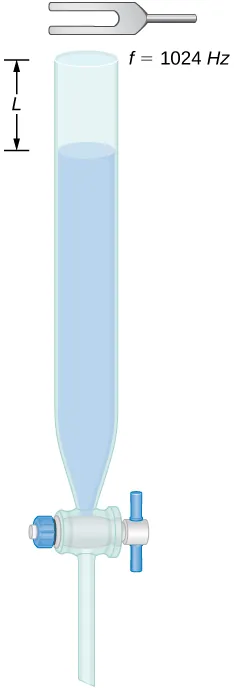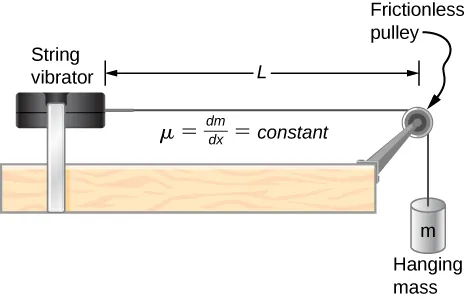University Physics Volume 1

129.

A 0.80-m-long tube is opened at both ends. The air temperature is $26°C.26°C.$ The air in the tube is oscillated using a speaker attached to a signal generator. What are the wavelengths and frequencies of first two modes of sound waves that resonate in the tube?

130.

A tube filled with water has a valve at the bottom to allow the water to flow out of the tube. As the water is emptied from the tube, the length L of the air column changes. A 1024-Hz tuning fork is placed at the opening of the tube. Water is removed from the tube until the $n=5n=5$ mode of a sound wave resonates. What is the length of the air column if the temperature of the air in the room is $18°C?18°C?$131.

Consider the following figure. The length of the string between the string vibrator and the pulley is $L=1.00m.L=1.00m.$ The linear density of the string is $μ=0.006kg/m.μ=0.006kg/m.$ The string vibrator can oscillate at any frequency. The hanging mass is 2.00 kg. (a)What are the wavelength and frequency of $n=6n=6$ mode? (b) The string oscillates the air around the string. What is the wavelength of the sound if the speed of the sound is $vs=343.00m/svs=343.00m/s$?132.

Early Doppler shift experiments were conducted using a band playing music on a train. A trumpet player on a moving railroad flatcar plays a 320-Hz note. The sound waves heard by a stationary observer on a train platform hears a frequency of 350 Hz. What is the flatcar’s speed in mph? The temperature of the air is $TC=22°CTC=22°C$.

133.

Two cars move toward one another, both sounding their horns $(fs=800Hz)(fs=800Hz)$. Car A is moving at 65 mph and Car B is at 75 mph. What is the beat frequency heard by each driver? The air temperature is $TC=22.00°CTC=22.00°C$.

134.

Student A runs after Student B. Student A carries a tuning fork ringing at 1024 Hz, and student B carries a tuning fork ringing at 1000 Hz. Student A is running at a speed of $vA=5.00m/svA=5.00m/s$ and Student B is running at $vB=6.00m/s.vB=6.00m/s.$ What is the beat frequency heard by each student? The speed of sound is $v=343.00m/s.v=343.00m/s.$

135.

Suppose that the sound level from a source is 75 dB and then drops to 52 dB, with a frequency of 600 Hz. Determine the (a) initial and (b) final sound intensities and the (c) initial and (d) final sound wave amplitudes. The air temperature is $TC=24.00°CTC=24.00°C$ and the air density is $ρ=1.184kg/m3.ρ=1.184kg/m3.$

136.

The Doppler shift for a Doppler radar is found by $f=fR(1+vc1−vc)f=fR(1+vc1−vc)$, where $fRfR$ is the frequency of the radar, f is the frequency observed by the radar, c is the speed of light, and v is the speed of the target. What is the beat frequency observed at the radar, assuming the speed of the target is much slower than the speed of light?

137.

A stationary observer hears a frequency of 1000.00 Hz as a source approaches and a frequency of 850.00 Hz as a source departs. The source moves at a constant velocity of 75 mph. What is the temperature of the air?

138.

A flute plays a note with a frequency of 600 Hz. The flute can be modeled as a pipe open at both ends, where the flute player changes the length with his finger positions. What is the length of the tube if this is the fundamental frequency?

Order a print copy

As an Amazon Associate we earn from qualifying purchases.